# What exactly does the Work-Energy theorem say?

• pkc111

#### pkc111

Homework Statement
State the work-energy theorem.
Relevant Equations
see below
My research leads to 2 slightly different equations. See equations 1 and 2 attached.
Also, for equation 1 should Wext be Wnet ext ?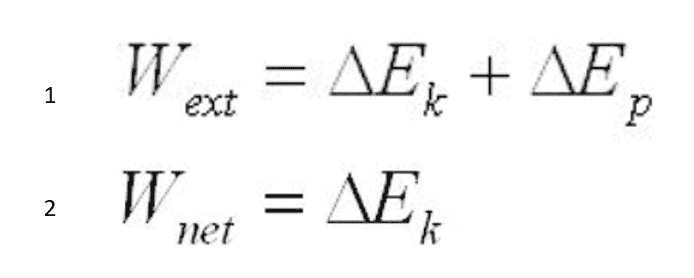Hi,
Homework Statement:: State the work-energy theorem.
Relevant Equations:: see below

My research leads to 2 slightly different equations. See equations 1 and 2 attached.
Also, for equation 1 should Wext be Wnet ext ?
View attachment 283156
Does your 'research' (any references ?) also have a context explaining what is meant with each of these variables ?

##\ ##

W ext = work done by external forces.
Ek = kinetic energy
Ep - potential energy
W net = net work

Point takenRemarkable that one can achieve a PhD in physics without knowing the work-energy theorem, isn't it . . . !

I do know, however, the terms and the equations (my question was an in vitation to you to think about what the symbols represent), so I still feel qualified to help.

Both your number 1 and number 2 are good candiates -- as your research shows. Since it's only a label, the homework statement would be better in place in an english class than in physics as far as I'm concerned. The physics point is that one understands what needs to be considered in a particular situation (hence my question about context: what is considered 'the system' determines what is internal and what is external. The presence or absence of of a conservative force field determines if mechanical energy conservation is expressed as nr 1 or as nr 2).

As a physicist I prefer number 1, but I see number 2 popping up quite often as well -- in the implicitly or explicitly mentioned context of the absence of a gravitational field.

Also, for equation 1 should Wext be Wnet ext ?
is a lot easier: yes!

##\ ##

•DaveE, Delta2, Hamiltonian and 3 others
Remarkable that one can achieve a PhD in physics without knowing the work-energy theorem, isn't it . . . !
Guess the OP expects us to have a PhD in clairvoyance. Since when do people have to explain their formulas?
Maybe we should add to our rules, that a good counterquestion is often half the way to the answer.

•BvU
it is a very frequent mistake: to take into account only the external forces in the work energy theorem. Actually the notion external\ internal is not relevant here

Perhaps it would be useful to formulate the work energy theorem here.
Assume we have a system of particles ##m_1,\ldots,m_N## with position vectors ##r_1,\ldots,r_N## relative an inertial frame. A net force that the particle ##m_i## experiences is presented as follows
$$F_i-\frac{\partial V}{\partial r_i},\quad V=V(r_1,\ldots,r_N).$$
And $$T=\frac{1}{2}\sum_{i=1}^Nm_i|\dot r_i|^2.$$
Theorem:
$$\frac{d}{dt}(T+V)= \sum_{i=1}^N (F_i,\dot r_i).$$

Last edited:
•Delta2
Thanks for the answers but I am still a bit confused.

Does anybody have a good link to the definition of the work-energy theorem and the conditions under which each equation is valid eg. presence or absence of conservative forces, whether the forces are external or internal? Do all the forces acting have to be conservative for these equations to work or just one of them?

Also just so just to confirm I understand, If I were to raise an apple vertically (1N) 1m with my hand at constant speed then I have done 1J of work on the apple, gravity has done -1J of work and the net work done on the apple is 0J? and if I consider the apple/earth the system, is my hand an external force and the weight an internal force? and does my hand apply a conservative or a nonconservative force?

OK so here is my latest attempt, criticism is welcome :)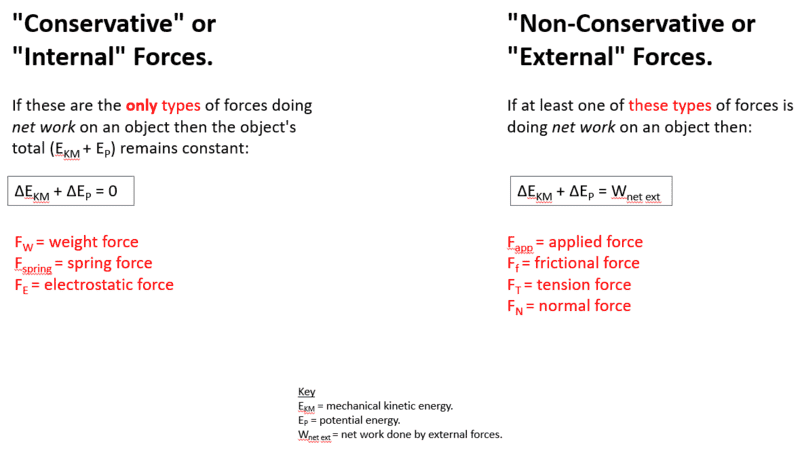Last edited:
•Delta2
again any criticism welcome..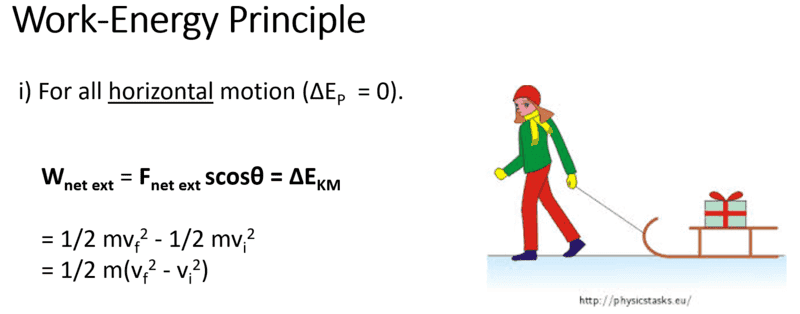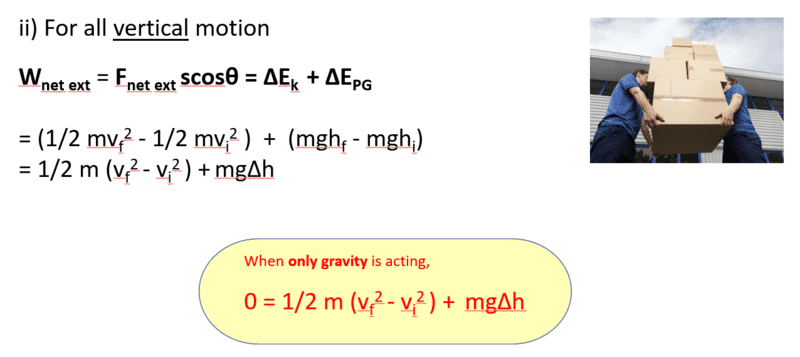What you saying in post #8 and #9 seems mostly correct. Just two things:
1. Post #8 seems to consider conservative forces as synonym for internal and non conservative as synonym for external. This is not completely correct, we can have a non conservative force that it is internal, for example take two bodies that touch. The friction in the touching area is a non conservative force and it is internal.
2. We can derive all this by the work energy theorem (##W_{net}=\Delta E_K##) and the definition of potential energy as the work done by the internal (or conservative) forces (##W_{int}=-\Delta E_P##).

Thanks Delta 2 that's very helpful :)

Just a question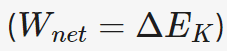So this one always for nonconservative forces or all forces? and the other equation just for conservative forces?

Thanks Delta 2 that's very helpful :)

Just a question
View attachment 283194
So this one always for nonconservative forces or all forces? and the other equation just for conservative forces?
This is for the work of all forces, conservative and non conservative. The other equation is just the definition of potential energy and it is only for conservative forces.
If you wonder how we derive it , here it is:
$$W_{net}=\Delta E_K\Rightarrow W_{net,int}+W_{net,ext}=\Delta E_K\Rightarrow -\Delta E_P+W_{net,ext}=\Delta E_K\Rightarrow W_{net,ext}=\Delta E_K+\Delta E_P$$

•